# Precalculus : Solve and Graph Polynomial Inequalities

## Example Questions

### Example Question #1 : Solving Polynomial And Rational Inequalities

What is the solution to the following inequality?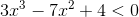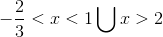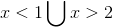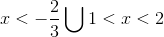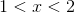Explanation:

First, we must solve for the roots of the cubic polynomial equation.

We obtain that the roots are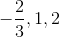.

Now there are four regions created by these numbers:

•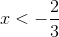. In this region, the values of the polynomial are negative (i.e.plug in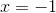and you obtain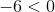•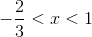. In this region, the values of the polynomial are positive (when, polynomial evaluates to)

•. In this region the polynomial switches again to negative.

•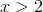. In this region the values of the polynomial are positive

Hence the two regions we want areand.

### All Precalculus Resources